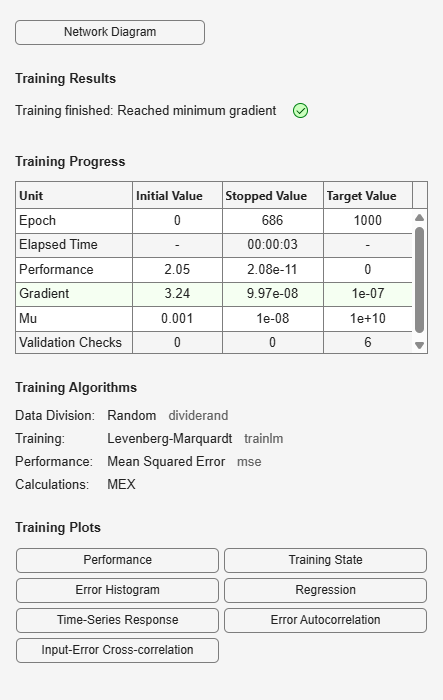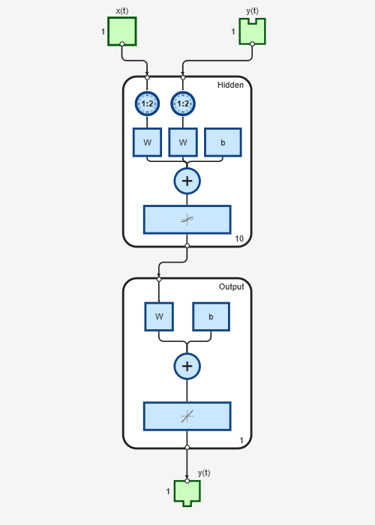# openloop

Convert neural network closed-loop feedback to open loop

## Syntax

```net = openloop(net) [net,xi,ai] = openloop(net,xi,ai) ```

## Description

`net = openloop(net)` takes a neural network and opens any closed-loop feedback. For each feedback output `i` whose property `net.outputs{i}.feedbackMode` is `'closed'`, it replaces its associated feedback layer weights with a new input and input weight connections. The `net.outputs{i}.feedbackMode` property is set to `'open'`, and the `net.outputs{i}.feedbackInput` property is set to the index of the new input. Finally, the value of `net.outputs{i}.feedbackDelays` is subtracted from the delays of the feedback input weights (i.e., to the delays values of the replaced layer weights).

`[net,xi,ai] = openloop(net,xi,ai)` converts a closed-loop network and its current input delay states `xi` and layer delay states `ai` to open-loop form.

## Examples

### Convert NARX Network to Open-Loop Form

This example shows how to create a NARX network in open-loop form, convert it to closed-loop form, and then convert it back.

```[X,T] = simplenarx_dataset; net = narxnet(1:2,1:2,10); [Xs,Xi,Ai,Ts] = preparets(net,X,{},T); net = train(net,Xs,Ts,Xi,Ai);````view(net)````Yopen = net(Xs,Xi,Ai); net = closeloop(net); view(net)``````[Xs,Xi,Ai,Ts] = preparets(net,X,{},T); Yclosed = net(Xs,Xi,Ai); net = openloop(net); view(net)``````[Xs,Xi,Ai,Ts] = preparets(net,X,{},T); Yopen = net(Xs,Xi,Ai);```

### Convert Delay States

For examples on using `closeloop` and `openloop` to implement multistep prediction, see `narxnet` and `narnet`.

## Version History

Introduced in R2010b